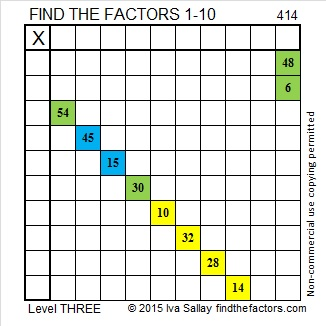# 414 and Level 3

414 is even and 4 + 1 + 4 = 9. These two facts help us to know that 414 can be evenly divided by 2, 3, 6, and 9. Its other factors are listed below the puzzle.

Today’s puzzle is really three puzzles in one. Solve some or all of them – your choice.

• What are the missing factors?
• Can you fill in the rest of the multiplication table?
• What is the significance of the colors in the puzzle? Perhaps someone will type that answer in the comments because, otherwise, I’m not telling! (But you can look at puzzles 417 or 422 for more hints.)Print the puzzles or type the factors on this black and white excel file: 10 Factors 2015-03-02

• 414 is a composite number.
• Prime factorization: 414 = 2 x 3 x 3 x 23, which can be written 414 = 2 x (3^2) x 23
• The exponents in the prime factorization are 1, 2, and 1. Adding one to each and multiplying we get (1 + 1)(2 + 1)(1 + 1) = 2 x 3 x 2 = 12. Therefore 414 has exactly 12 factors.
• Factors of 414: 1, 2, 3, 6, 9, 18, 23, 46, 69, 138, 207, 414
• Factor pairs: 414 = 1 x 414, 2 x 207, 3 x 138, 6 x 69, 9 x 46, or 18 x 23
• Taking the factor pair with the largest square number factor, we get √414 = (√9)(√46) = 3√46 ≈ 20.3470This site uses Akismet to reduce spam. Learn how your comment data is processed.